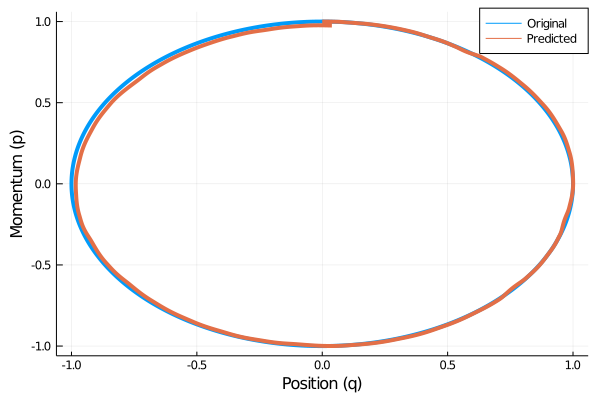# Hamiltonian Neural Network

Hamiltonian Neural Networks introduced in  allow models to "learn and respect exact conservation laws in an unsupervised manner". In this example, we will train a model to learn the Hamiltonian for a 1D Spring mass system. This system is described by the equation:

$$$m\ddot x + kx = 0$$$

Now we make some simplifying assumptions, and assign $m = 1$ and $k = 1$. Analytically solving this equation, we get $x = sin(t)$. Hence, $q = sin(t)$, and $p = cos(t)$. Using these solutions we generate our dataset and fit the NeuralHamiltonianDE to learn the dynamics of this system.

using Flux, DiffEqFlux, DifferentialEquations, Statistics, Plots, ReverseDiff

t = range(0.0f0, 1.0f0, length = 1024)
π_32 = Float32(π)
q_t = reshape(sin.(2π_32 * t), 1, :)
p_t = reshape(cos.(2π_32 * t), 1, :)
dqdt = 2π_32 .* p_t
dpdt = -2π_32 .* q_t

data = cat(q_t, p_t, dims = 1)
target = cat(dqdt, dpdt, dims = 1)

hnn = HamiltonianNN(
Chain(Dense(2, 64, relu), Dense(64, 1))
)

p = hnn.p

loss(x, y, p) = mean((hnn(x, p) .- y) .^ 2)

callback() = println("Loss Neural Hamiltonian DE = $(loss(data, target, p))") epochs = 500 for epoch in 1:epochs for (x, y) in dataloader gs = ReverseDiff.gradient(p -> loss(x, y, p), p) Flux.Optimise.update!(opt, p, gs) end if epoch % 100 == 1 callback() end end callback() model = NeuralHamiltonianDE( hnn, (0.0f0, 1.0f0), Tsit5(), save_everystep = false, save_start = true, saveat = t ) pred = Array(model(data[:, 1])) plot(data[1, :], data[2, :], lw=4, label="Original") plot!(pred[1, :], pred[2, :], lw=4, label="Predicted") xlabel!("Position (q)") ylabel!("Momentum (p)") ## Step by Step Explanation ### Data Generation The HNN predicts the gradients$(\dot q, \dot p)$given$(q, p)$. Hence, we generate the pairs$(q, p)$using the equations given at the top. Additionally to supervise the training we also generate the gradients. Next we use use Flux DataLoader for automatically batching our dataset. using Flux, DiffEqFlux, DifferentialEquations, Statistics, Plots, ReverseDiff t = range(0.0f0, 1.0f0, length = 1024) π_32 = Float32(π) q_t = reshape(sin.(2π_32 * t), 1, :) p_t = reshape(cos.(2π_32 * t), 1, :) dqdt = 2π_32 .* p_t dpdt = -2π_32 .* q_t data = cat(q_t, p_t, dims = 1) target = cat(dqdt, dpdt, dims = 1) dataloader = Flux.Data.DataLoader((data, target); batchsize=256, shuffle=true) MLUtils.DataLoader{Tuple{Matrix{Float32}, Matrix{Float32}}, Random._GLOBAL_RNG, Val{nothing}}((Float32[0.0 0.006141883 … -0.006141926 1.7484555f-7; 1.0 0.99998116 … 0.99998116 1.0], Float32[6.2831855 6.283067 … 6.283067 6.2831855; -0.0 -0.038590588 … 0.03859086 -1.0985871f-6]), 256, false, true, true, false, Val{nothing}(), Random._GLOBAL_RNG()) ### Training the HamiltonianNN We parameterize the HamiltonianNN with a small MultiLayered Perceptron (HNN also works with the Fast* Layers provided in DiffEqFlux). HNNs are trained by optimizing the gradients of the Neural Network. Zygote currently doesn't support nesting itself, so we will be using ReverseDiff in the training loop to compute the gradients of the HNN Layer for Optimization. hnn = HamiltonianNN( Chain(Dense(2, 64, relu), Dense(64, 1)) ) p = hnn.p opt = ADAM(0.01) loss(x, y, p) = mean((hnn(x, p) .- y) .^ 2) callback() = println("Loss Neural Hamiltonian DE =$(loss(data, target, p))")

epochs = 500
for epoch in 1:epochs
gs = ReverseDiff.gradient(p -> loss(x, y, p), p)
Flux.Optimise.update!(opt, p, gs)
end
if epoch % 100 == 1
callback()
end
end
callback()
Loss Neural Hamiltonian DE = 16.441914
Loss Neural Hamiltonian DE = 0.021214506
Loss Neural Hamiltonian DE = 0.012675277
Loss Neural Hamiltonian DE = 0.008989096
Loss Neural Hamiltonian DE = 0.007957665
Loss Neural Hamiltonian DE = 0.0078066066

### Solving the ODE using trained HNN

In order to visualize the learned trajectories, we need to solve the ODE. We will use the NeuralHamiltonianDE layer which is essentially a wrapper over HamiltonianNN layer and solves the ODE.

model = NeuralHamiltonianDE(
hnn, (0.0f0, 1.0f0),
Tsit5(), save_everystep = false,
save_start = true, saveat = t
)

pred = Array(model(data[:, 1]))
plot(data[1, :], data[2, :], lw=4, label="Original")
plot!(pred[1, :], pred[2, :], lw=4, label="Predicted")
xlabel!("Position (q)")
ylabel!("Momentum (p)")## Expected Output

Loss Neural Hamiltonian DE = 18.768814
Loss Neural Hamiltonian DE = 0.022630047
Loss Neural Hamiltonian DE = 0.015060622
Loss Neural Hamiltonian DE = 0.013170851
Loss Neural Hamiltonian DE = 0.011898238
Loss Neural Hamiltonian DE = 0.009806873

 Greydanus, Samuel, Misko Dzamba, and Jason Yosinski. "Hamiltonian Neural Networks." Advances in Neural Information Processing Systems 32 (2019): 15379-15389.